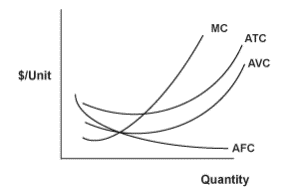# Marginal Revenue and Marginal Cost

• Marginal Analysis:

Is an exercise that helps a company make decisions to maximize their profits by comparing the additional benefits and the additional costs generated by increasing their output of the same activity.

• When Marginal Benefit > Marginal Costs, the company should increase the activity output.
• When Marginal Benefit < Marginal Costs, the company should cut down on the output.

• Marginal Revenue (MR):

It measures the increase/decrease in revenue for producing and selling one more unit of item.

• MR = ΔTR/ΔQ

Where TR= Total Revenue

• Marginal Cost (MC):

It measures the increase/decrease in total cost of producing one more unit of an item.
The formula used to calculate marginal cost is as follow:

• MC = ΔTC/ΔQ

Where TC= Total Cost and Q= Quantity.

• Profit Maximization (Marginal Profit):

It occurs when Marginal revenue = Marginal costs. Any points after ‘Profit Maximization’, will cause the prices to rise and gradually diminish the profit (Marginal Loss).

Note: company should always target to increase its profitsnot its revenue.

• Marginal Cost (MC) and Average Total Cost (ATC):
• Total Cost of production (TC) = Fixed Costs (FC) + Variable Costs (VC).
• Average Total Costs (ATC)= Total cost/Q
• Average Fixed Costs (AFC)= fixed cost/Q
• Average Variable Costs (AVC) = variable cost/Q

When an increase occurs in relation to Fixed cost, the:

1. FC and AFC increases.
2. TC and ATC increases.
3. VC and AVC will have no effect.ATC

MC always interconnect with ATC & AVC at their lowest points for the short run.

Why companies do these Analysis:

• When Marginal Revenue < Marginal cost= the company is over producing so it should decrease the quantity supplied.
• When Marginal Revenue > Marginal cost= the company is not producing enough so it should increase the quantity supplied.

0 replies